# SBI PO Reasoning Questions 2019 (Day-17) High Level-New Pattern

SBI PO 2019 Notification is about to come and it is the most awaited exam among the aspirants. We all know that new pattern questions are introducing every year in the SBI PO exam. Further, the questions are getting tougher and beyond the level of the candidate’s expectations.

Our IBPS Guide is providing High-Level New Pattern Reasoning Ability Questions for SBI PO 2019 so the aspirants can practice it on a daily basis. These questions are framed by our skilled experts after understanding your needs thoroughly. Aspirants can practice these high-level questions daily to familiarize with the exact exam pattern. We wish that your rigorous preparation leads you to a successful target of becoming SBI PO.

[WpProQuiz 5134]

### Click Here for SBI PO Pre 2019 High-Quality Mocks Exactly on SBI Standard

(Directions 1–2): Study the following information carefully and answer the questions given below it.

^ – South

% – East

@ – North

# – West

\$ – Either 2 metre or 3 metre.

£ – Either 4 metre or 5 metre.

i.e., P ^ Q means P is south of Q.

P ^% Q means P is South-East of Q.

P ^\$ Q means P is either 2 metre or 3 metre south of Q.

(i) H ^\$ G

(ii) F @# E

(iii) B #\$ C

(iv) A # H

(v) F %\$ G

(iv) E %£ D

(vii) D @£ C

(viii) G @ C

(ix) E £ F

(x) A @ B

(xi)Distance between EF is more than CD.

(xii) Distance between BC is more than FG.

(xiii) Distance between CG is equal to 8m.

(xiv) Distance between (AH + GH) = ED.

1) What is the position of A with respect B?

a) 7m, North

b) 5m, North

c) 4m, North

d) 6m, North

e) Can’t be determined.

2) What is the position of F with respect A?

a) North-west

b) North-East

c) South-East

d) East

e) Can’t be determined.

(Directions 3-5): A string of numbers is given as input. The further steps given are obtained by applying certain logic. Each step is a resultant of previous step only. Study the following information carefully and answer the questions given below it.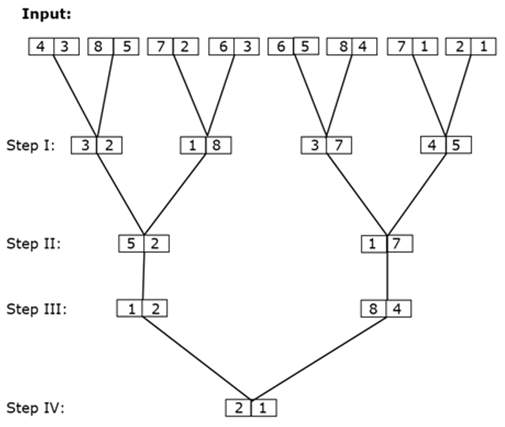As per above applied logic in above steps, find appropriate step for given input: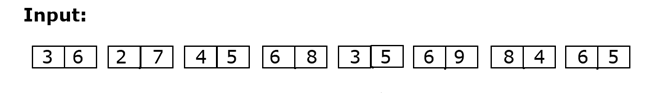3) What is sum of digits of lowest numbers obtained in step III?

a) 12

b) 7

c) 9

d) 13

e) None of these

4) Which of the following number is second lowest in step I?

a) 37

b) 23

c) 18

d) 27

e) None of these

5) What is the difference of square of number in step II?

a) 440

b) 388

c) 240

d) 360

e) None of these

Directions (6-10): Study the following information carefully and answer the below questions.

There is a board in the form of 5×7 matrix in which rows are labeled as A, B, C, D & E from top to bottom and columns are labeled as @, %, &, #, ¥, ^ and £ from right to left. All consecutive odd numbers are written in each cell from left to right in each row such that ‘A£’ has smallest odd number and ‘[email protected]’ has the largest possible odd number. Then the cells in the matrix are filled with respect to the below statements:

Note: The letters of the word ‘JOURNEY’ is filled first before filling the letters of the word ‘PLASTIC’. Each cell should either contains one number and letter or one number and symbol

• All the letters of the word “JOURNEY” are written in alphabetical order to each cell having multiple of 5.
• All the letters of the word “PLASTIC” are written in reverse alphabetical order to each of the remaining cell having number multiple of 3.
• ‘%’ is written to each remaining cells which are preceded by a vowel in a row.
• ‘K’ is immediately preceded by ‘&’. One element is written between ‘K’ & ‘Y’.
• Two elements are written between ‘@’ and ‘#’ in the same row. ‘@’ is written left of ‘#’, which is not written in same column having ‘U’.
• ‘*’ and ‘G’ are written in the same row. ‘β’ is preceded by ‘D’. ‘%’ and ‘F’ are written in same column.
• ‘Z’ and ‘Q’ are not written in same column. Also ‘Z’ and ‘Y’ are not written in the same row.
• ‘G’ and ‘M’ are written in the same column next to each other.
• ‘M’ is followed by ‘¥’.
• At least four and at most eight elements are written between ‘B’ and ‘Z’. B is written before Z.
• ‘B’ is preceded by ‘\$’. ‘G’ is preceded by ‘£’.
• ‘Q’ is written before ‘A’ but not in any cell having prime number. Q and A are not written in the same row.
• ‘V’ and ‘W’ are written in same column.
• ‘G’ is written after ‘M’. ‘D’ and ‘V’ are written in same row.

6) What is the correct cell position of ‘V’?

a) E^

b) D¥

c) E&

d) C£

e) None of these

7) Four out of five are related to each other in some way and thus form a group, then which of the following doesn’t belongs to the group?

a) MZJ

b) W%R

c) Vβ&

d) E\$T

e) G%£

8) Which of the following element is in the adjacent cell of ‘W’?

a) #

b) @

c) A

d) F

e) None of these

9) Which of the following element is present in the ‘E%’ cell of the matrix?

a) 67K

b) 53%

c) 35O

d) 67&

e) None of these

10) Which of the following statement is/are true?

a) The cell ‘B#’ of the matrix is written as ‘21Z’

b) ‘31G’ is written in the ‘D^’ cell of the matrix.

c) The cell ‘E¥’ of the matrix is written as ‘61V’

d) ‘65F’ is written in the ‘E&’ cell of the matrix.

e) All are true

(Directions 1–2):

We have:

• H is either 2m or 3m south of G.
• F is north-west of E.
• B either 2m or 3m west of C.
• A is west of H.
• F is either 2m or 3m east of G.
• E is either 4m or 5m east of D.
• D is either 4m or 5m north of C.
• G is north of C.
• A is north of B.

Based on above given information we have: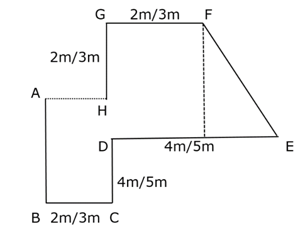Again, we have:

• Distance between EF is more than CD, that means EF = 5m and CD = 4m.
• Distance between BC is more than FG, that means BC = 3m and FG = 2m.
• Distance between (AH + GH) = ED, that means AH = 3m.
• Distance between CG is equal to 8m, as EF is hypotenuse for triangle formed by a perpendicular drawn from F on DE.

Since, EF = 5m, then perpendicular must be 4 meter.

Thus, to satisfies all the given of GC = 8m.

DC = 4m.

Thus, possible combination of length of GD must be (2m, 2m)

Based on above given information we have final arrangement as follow: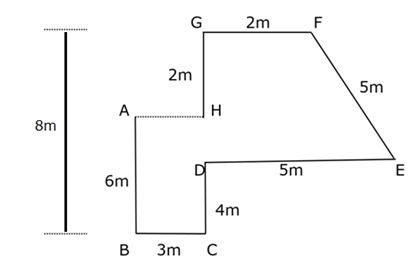Clearly, A is 6m north of B.

Hence, option D is correct choice.

Clearly, F is north-east of A.

Hence, option B is correct choice.

(Directions 3-5):

We have given input: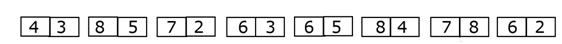From above logical steps we get following results for given input:

Step I: In this step following logic is applied: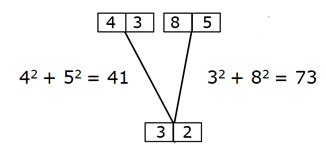Final result can be determined by difference = (73 – 41) = 32

Step II: In this step following logic is applied: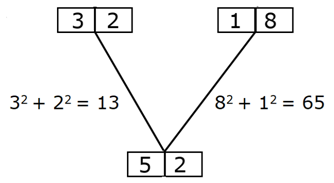Final result can be determined by difference = (65 – 13) = 52

Step III: In this step following logic is applied: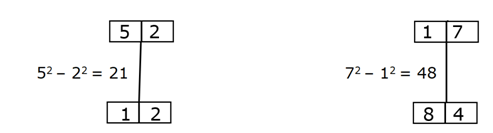Final result can be determined by interchanging digits of result.

Step IV: In this step following logic is applied: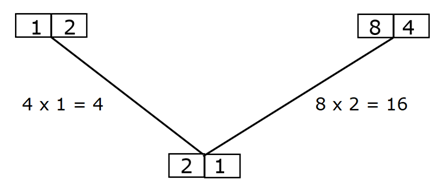Difference = (16 – 4) = 12.

Final result can be determined by interchanging digits of result obtained.

From above logical steps we get following results for given input: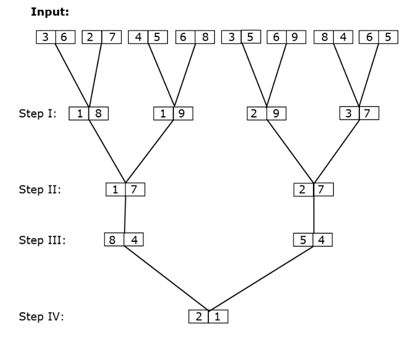Required sum of digits = (5 + 4) = 9

Hence, option C is correct choice.

Clearly, 19 is second lowest in step I.

Hence, option E is correct choice.

Clearly, required difference = (272 – 172) = 440

Hence, option A is correct choice.

Directions (6-10):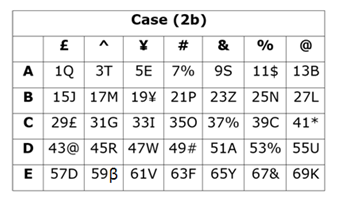We have:

• A 5×7 matrix in which rows are labeled 1 to 5 from top to bottom and columns are labeled as @, %, &, #, ¥, ^ and £ from right to left.
• All consecutive odd numbers are written in each cell from left to right in each row such that ‘1£’ has smallest odd number and ‘[email protected]’ has the largest possible odd number.
• All the letters of the word “JOURNEY” are written in alphabetical order to each cell having multiple of 5.
• All the letters of the word “PLASTIC” are written in reverse alphabetical order to each remaining cell having number multiple of 3.

Based on above given information we have: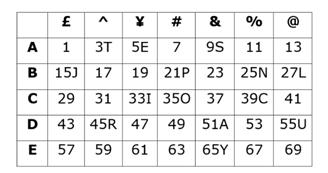Again, we have:

• ‘%’ is written to each remaining cell which are preceded by a vowel.
• One element is written between ‘K’ & ‘Y’, that means we have two possible position for ‘K’, in case (1) K is written in cell ‘E¥’, in case (2) K is written in cell ‘[email protected]’.
• ‘K’ is immediately preceded by ‘&’.
• Two elements are written ‘@’ and ‘#’ in the same row.
• ‘@’ is written left of ‘#’, which is not written in same column having ‘U’, that means we have six possibility for @, in case (1a)& case (2a)‘@’ is written in ‘B^’, in case (1b)& case (2b)‘@’ is written in ‘D£’, in case (1c)& case (2c) ‘@’ is written in ‘E£’.

Based on above given information we have: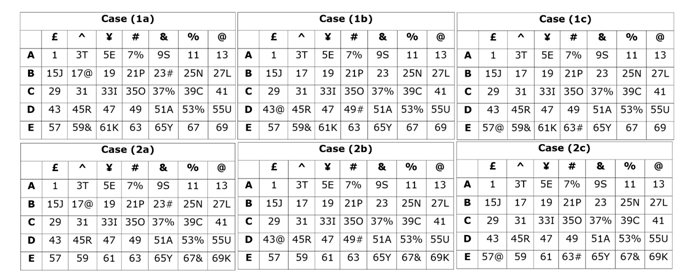Again, we have:

• ‘G’ is preceded by ‘£’.
• ‘*’ and ‘G’ are written in the same row.
• ‘G’ and ‘M’ are written in the same column next to each other.
• ‘G’ is written after ‘M’.
• ‘M’ is followed by ‘¥’, that means in case (1b), case (1c), case (2b) & case (2c) G written in the cell ‘C^’, and case (1a) & case (2a) are not valid.

Based on above given information we have: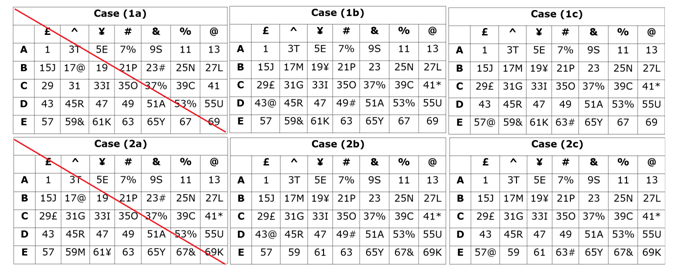Case (2a) is not valid as ‘M’ is written after ‘G’.

Again, we have:

• ‘Q’ is written before ‘A’ but not in any cell having prime number. Q and A are not written in the same row,that means ‘Q’ is written at ‘A£’.
• At least four and at most eight elements are written between ‘B’ and ‘Z’. B is written before Z.
• ‘B’ is preceded by ‘\$’.
• ‘Z’ and ‘Q’ are not written in same column. Z and Y are not written in the same row.
• ‘V’ and ‘W’ are written in same column.
• ‘D’ and ‘V’ are written in same row.
• ‘β’ is preceded by ‘D’, that means case (1b), case (1c) & case (2c) are not valid and in case (2b) V is written ‘E¥’.
• ‘%’ and ‘F’ are written in same column which means in case (2b) F is written in the cell ‘E #’.

Based on above given information we have final arrangement as follow: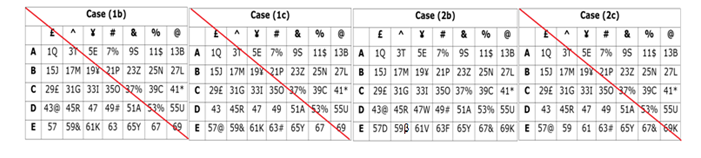Case (1b), case (1c) & case (2c) are not valid as ‘V’ and ‘W’ are written in the same column.

Clearly, correct cell position of ‘V’ is ‘E¥’.

Hence, option E is the correct choice.

Clearly, in each combination 2nd element is second to right of 1stelement and 3rd element is immediate left of 1st element.

Thus, “Vβ&” doesn’t belong to same group.

Hence, option C is the correct choice.

Clearly, ‘#’ is immediate right of W.

Hence, option A is the correct choice.

Clearly, 67& is at ‘E%’

Hence, option D is the correct choice.